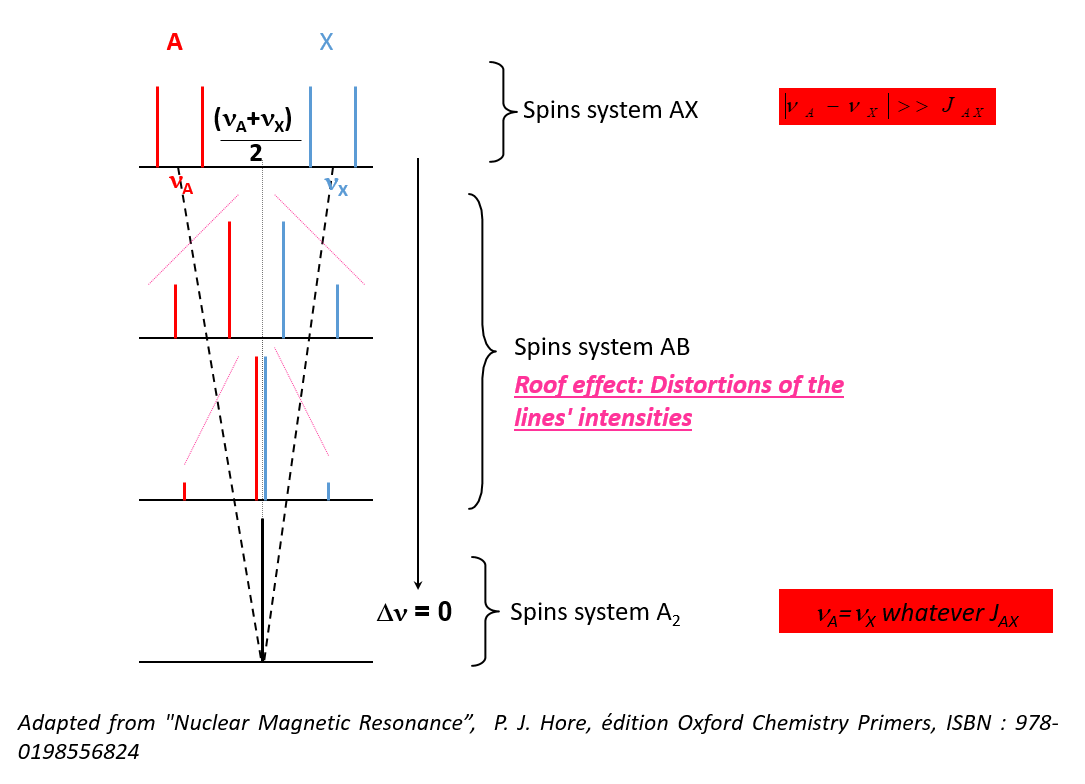## First order or second order spectraWhen the non-secular term has a negligible contribution compared to secular terms, the spin system is of the first order: The rules of multiplicity of Pascal's binomial apply in this case only.

• In order to detect whether a signal is of the first or second order, the following quantity must be evaluated:• Approximation of weakly coupled nuclei: :I and J are then written A and X, the spectrum is of the first order
• If this condition is not respected, the nuclei are said to be strongly coupled• Changes in the intensity of the lines
• Changes in the number of lines
• Spectral analysis requires theoretical calculations

Spectral analysis requires the simulation of NMR spectra

Consequences:
• It is no longer possible to measure J directly (except in the simple case AB)
• Source of error in the interpretation of the spectra
• Spectra analysis request calculation
Possible solution:
• Analyze the sample with a higher magnetic field
• Δν depends on B0
• J is independent of B0### Isochrony

• Two nuclei are CHEMICALLY EQUIVALENT, if they have the same chemical environment, that is if geometrically there is at least one element of symmetry (plane, axis, center) between them.
• If the symmetry element is at least one proper axis of symmetry, the nuclei are said to be homotopic
• If the symmetry element is strictly an improper axis of symmetry (center, plane, axis of rotation-reflection), the nuclei are called enantiotopes
The two nuclei therefore have the same NMR resonance frequency: they are ISOCHRONES

### Isogamy

• Two nuclei are ISOGAMIC if they have the same coupling relationships with any of their neighbors that do not have the same chemical shift.
Remarks:
• Two nuclei cannot be isogamic if they are not at least isochronous
• It makes no sense to test the isogamy of a pair of nuclei if they do not have at least the same chemical environment. Indeed, in this case, they necessarily already have different coupling relationships with a given third party!

### Chemical and magnetic equivalence

• Two CHEMICALLY EQUIVALENT nuclei are ISOCHRONOUS
• Two MAGNETICALLY EQUIVALENT nuclei are ISOCHRONOUS & ISOGAMIC
Corollaries:
• Two nuclei can therefore be chemically, but not magnetically, equivalent if they are not isogamic.
• Two magnetically equivalent nuclei are necessarily chemically equivalent

### Nomenclature of spin systems

• Nuclei that have no equivalence relations are written with different letters
Example: AX, AMX…
• Magnetically equivalent nuclei or clusters of nuclei are written with the same letter, indicated by the number of nuclei involved in each group
Example: A2, X2, X6, A3X2, AMX2, ...
• Chemically equivalent nuclei or clusters of nuclei are written with the same letter, but are differentiated from each other by a typographic character. There are as many characters as there are spins involved in the group considered
Example: AA’, XX’, AA’KX3, AA’A ’’A’’’A(4)A(5)A(6)...Next page
Previous page